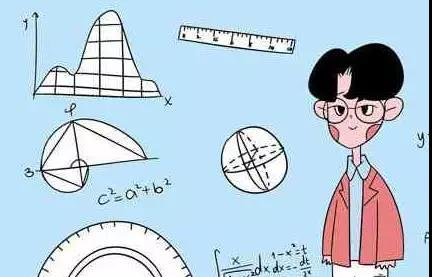# 小学数学加（+）减（-）乘（×）除（ ÷） 法速算技巧！15×17

15 + 7 = 22
5 × 7 = 35
---------------
255

15×17
=15 ×（10 + 7）
=15 × 10 + 15 × 7
=150 + （10 + 5）× 7
=150 + 70 + 5 × 7

=（150 + 70）+（5 × 7）

17 + 9 = 26
7 × 9 = 63

51 × 31
50 × 30 = 1500
50 + 30 = 80

------------------
1580

81 × 91
80 × 90 = 7200
80 + 90 = 170

------------------
7370
------------------
7371

43 × 46
（43 + 6）× 40 = 1960
3 × 6 = 18
----------------------
1978

（89 + 7）× 80 = 7680
9 × 7 = 63
----------------------

7743

56 × 54
(5 + 1) × 5 = 30--
6 × 4 = 24
----------------------
3024

(7 + 1) × 7 = 56--
3 × 7 = 21

----------------------
5621

(2 + 1) × 2 = 6--
1 × 9 = 9
----------------------
609

“--”代表十位和个位，因为两位数的首位相乘得数的后面是两个零，请大家明白，不要忘了，这点是很容易被忽略的。

56 × 58
5 × 5 = 25--
（6 + 8 ）× 5 = 7--

6 × 8 = 48
----------------------
3248

66 × 37
（3 + 1）× 6 = 24--
6 × 7 = 42
----------------------
2442

99 × 19
（1 + 1）× 9 = 18--
9 × 9 = 81
----------------------
1881

46 × 99
4 × 9 + 9 = 45--
6 × 9 = 54
-------------------
4554

82 × 33
8 × 3 + 3 = 27--
2 × 3 = 6
-------------------
2706

78 × 38
7 × 3 + 8 = 29--
8 × 8 = 64
-------------------
2964

23 × 83
2 × 8 + 3 = 19--

3 × 3 = 9

--------------------
190917 × 17
17 ＋ 7 = 24-
7 × 7 = 49
---------------
289

71 × 71
7 × 7 = 49--
7 × 2 = 14-
-----------------
5041

35 × 35
（3 + 1）× 3 = 12--
25
----------------------

1225

21 × 21 = 441
22 × 22 = 484
23 × 23 = 529
24 × 24 = 576

37 × 37
37 - 25 = 12--
（50 - 37）^2 = 169

----------------------

1369

26 × 26
26 - 25 = 1--
（50-26）^2 = 576
-------------------
6761、 被除数 ÷ 5
= 被除数 ÷ (10 ÷ 2)
= 被除数 ÷ 10 × 2
= 被除数 × 2 ÷ 10

2、 被除数 ÷ 25
= 被除数 × 4 ÷100
= 被除数 × 2 × 2 ÷100

3、 被除数 ÷ 125
= 被除数 × 8 ÷1000
= 被除数 × 2 × 2 × 2 ÷1000

# 素材来源网络，如涉及侵权，请联系删除！ / 声明 ▍